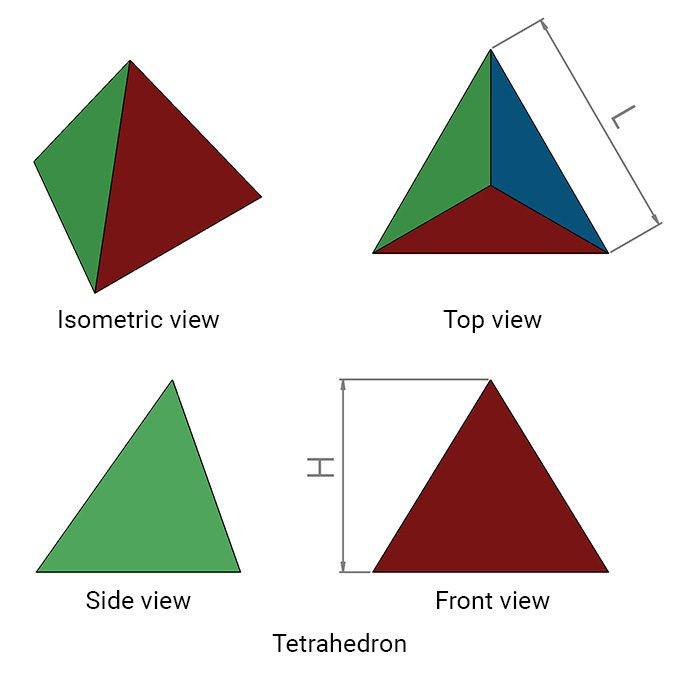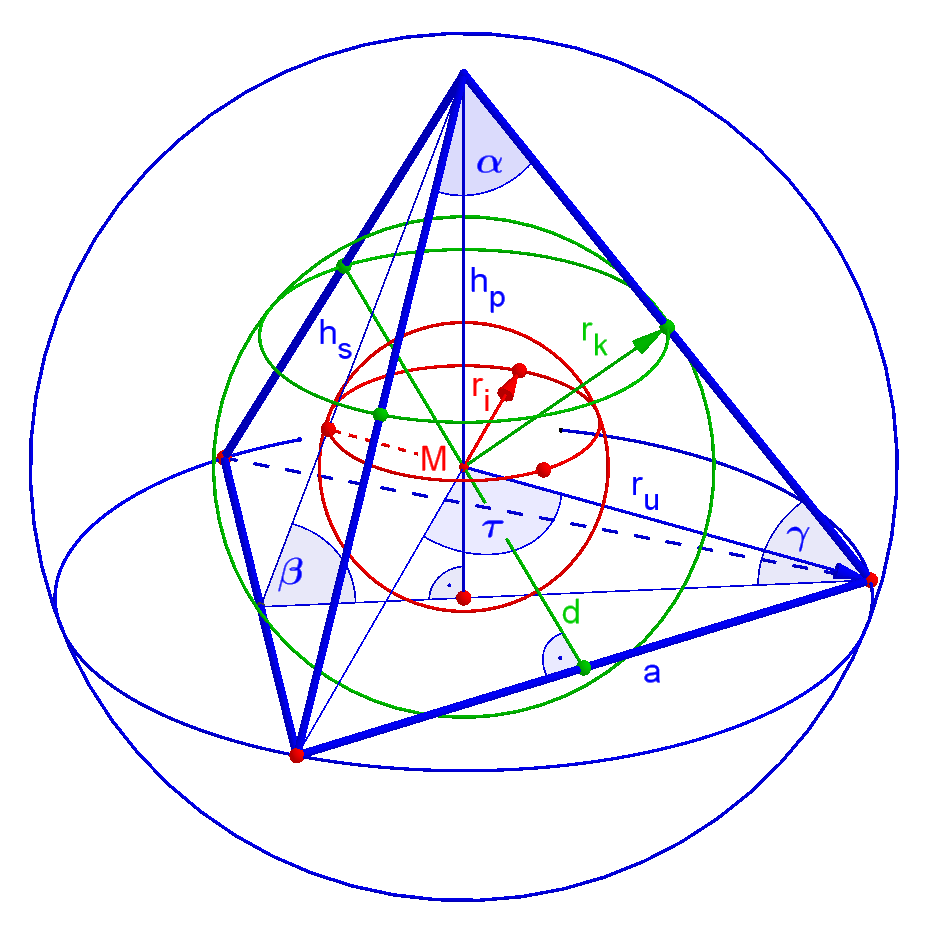# Tetrahedron Volume Calculator

Created by Rahul Dhari
Reviewed by Steven Wooding
Last updated: Dec 01, 2021

The tetrahedron volume calculator determines the volume and surface area of a tetrahedron. In addition to this, the calculator will also help you find other properties such as the height of tetrahedron, surface area to volume ratio, sizes of various spheres like insphere, midsphere, and circumsphere. Read on to understand the properties of a tetrahedron, how to calculate volume and surface area of a tetrahedron along with various spheres and how to find its surface area to volume ratio.

If you're not interested in the surface area to volume ratio, but in ratios in general, take a look at our ratio calculator!

## What is a tetrahedron? – Volume, surface area, and height of tetrahedron

A tetrahedron is a 3D shape that is formed by joining together 4 triangular faces. It has 4 vertices, 6 edges, and 4 faces. We can see different views of a tetrahedron in the figure below, with labels for height H and edge length L. This shape is otherwise known as a triangular pyramid, i.e., a pyramid with a triangular base.The height H of a tetrahedron is related to its edge length L can be written as:

H = (√6 / 3) * L

The volume of tetrahedron formula can be written as:

V = L3 / (6 * √2)

Similarly, the area of a tetrahedron A can be determined from the equation:

A = √3 * L2

You can observe that the surface area of the tetrahedron is the product of the number of faces and area of 1 triangular face. You can use the volume and surface area of the tetrahedron to estimate the surface area to volume ratio SVR for this shape.

Surface Area / Volume = (√3 * L2) / (L3 / (6 * √2))
SVR = 6 * √6 / L

## Spheres of a tetrahedron

Now that you know how to calculate volume and surface area, let us take a look at different spheres which can be accommodated inside and around a tetrahedron. There are three different kinds of spheres:

• Insphere – If you draw a sphere inside the tetrahedron which is tangent to every face of the cell, the sphere is known as an insphere. The calculator will estimate the largest possible size of the sphere that can be contained inside the faces of a tetrahedron with radius ri. The insphere is marked in red in the figure below.
ri = L / √24
• Midsphere – If you draw a sphere which is tangent to every edge of a tetrahedron, the sphere is called a midsphere. In other words, the sphere must touch every edge only at one point. The calculator will return the size of the midsphere having radius rk. The midsphere is marked in green in the figure below.
rk = L / √8
• Circumsphere – The sphere which touches the vertex of the tetrahedron is known as a circumsphere. The calculator determines the size of the circumsphere having radius ru. The circumsphere is marked in blue in the figure below.
ru = L * √6 / 4## How to use tetrahedron volume calculator?

Follow the steps below to determine various properties for your tetrahedron. As you can observe, the properties of a tetrahedron like surface area, volume, height, etc., are a function of only one variable, i.e., edge length, L. It is fairly easy to operate the tetrahedron volume calculator.

• Step 1: Enter the edge length L.
• Step 2a: The calculator will provide the height of the tetrahedron.
• Step 2b: The volume and surface area of the tetrahedron are also visible.
• Step 2c: The volume and surface area used to estimate the surface area to volume ratio.
• Step 2d: The sizes of insphere, midsphere, and circumsphere are also determined.

## Example: Using the tetrahedron volume calculator

Use the volume of tetrahedron formula to estimate the volume for the cell having edge length L = 80 cm. Also, find the sizes of insphere, midsphere, and circumsphere.

• Step 0: Set the units for edge length to cm.

• Step 1: Enter the edge length as 80 cm.

• Step 2a: The calculator will now return the volume:

Using the volume of tetrahedron formula,

V = L3 / (6 * √2)
V = 803 / (6 * √2) = 0.06034 m3
• Step 2b: The sizes for the sphere are calculated as:

Insphere:

ri = L /√24 = 80/√24 = 0.1633 m

Midsphere:

rk = L / √8 = 80 / √8 = 0.28284

Circumsphere:

ru = L * √6 / 4 = 80 * √6 / 4 = 0.4899

## Using tetrahedrons

Did you know:

• Tetrahedrons are used in complex stress and deformation-based computer simulations. Large shapes are segmented into smaller elements of tetrahedron shape.

• Tetrahedrons are also an observed shape of hybrid or bonded molecules in chemistry.

• Some of the ancient civilizations used tetrahedral dices for their board games. So does several current board games.

## FAQ

### What is a tetrahedron?

A tetrahedron is a 3D pyramidal shape with a triangular base.

### How do I calculate the volume of a tetrahedron?

The volume of tetrahedron formula can be calculated using the edge length, L as:

V = L3 / (6 * √2)

### How do I find the height of a tetrahedron?

The height of the tetrahedron can be calculated using the edge length, L as:

H = (√6 / 3) * L

### How many faces does a tetrahedron have?

A tetrahedron has 4 faces, 6 edges, and 4 vertices.

### How many edges does a tetrahedron have?

A tetrahedron has 6 edges and 4 vertices.

### How many vertices does a tetrahedron have?

A tetrahedron has 4 vertices.

Rahul Dhari
Edge length (L)
ft
Height of tetrahedron (H)
ft
Volume of tetrahedron (V)
cu in
Surface Area
Surface area of tetrahedron (A)
in²
Surface to volume ratio (SVR)
1/
in
Spheres of Tetrahedron
ft
ft
ft
People also viewed…

### Cylinder volume gallons

Use this cylinder volume gallons calculator to determine cylinder volume in gallons.

### Plastic Footprint

Find out how much plastic you use throughout a year with this plastic footprint calculator. Rethink your habits, reduce your plastic waste, and make your life a little greener.

### Snowman

The perfect snowman calculator uses math & science rules to help you design the snowman of your dreams!

### Triangle congruence

Are those two triangles the same? Find it out with our triangle congruence calculator!